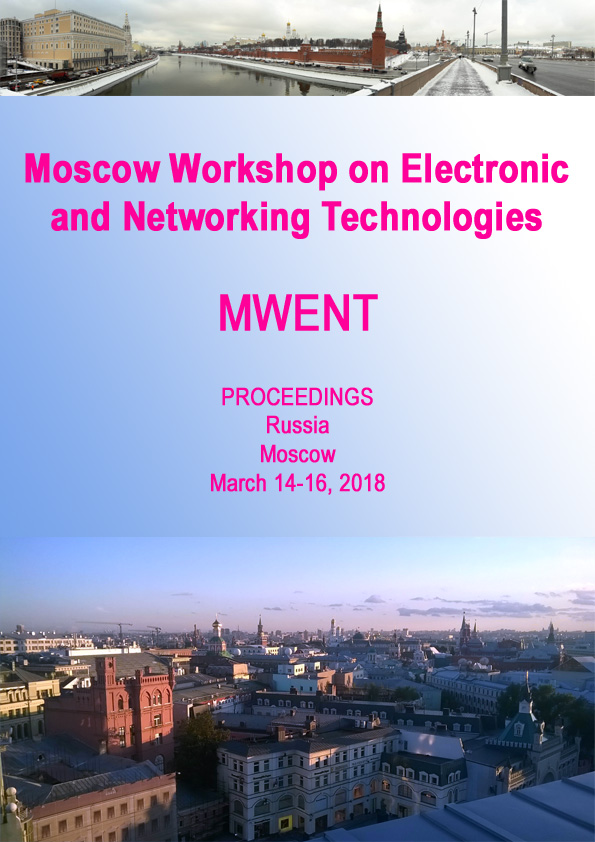• A
• A
• A
• ABC
• ABC
• ABC
• А
• А
• А
• А
• А
Regular version of the site

## Averaged Absolute Spectral Correlation Density Estimator

P. 1-4.
Shevgunov T., Efimov E., Zhukov D.

Introduced in this paper, the averaged absolute spectral correlation density estimator (AASCD) is based on time-smoothing algorithm of estimating spectral correlation density (SCD) using a long-time sample of discrete signal. The key feature of proposed estimator is compressing the cyclic frequency dimension of SCD estimation to make the matrix containing the SCD estimation cells balanced in both dimensions. The compressing is performed by means of averaging the absolute values of SCD in blocks of neighboring cyclic frequencies which prevents any components of a signal possessing wide-sense cyclostationarity from leaving out of the coverage of the estimator. The application of the proposed algorithm to the cyclic property analysis of the baseband signal in a digital interface measured by an oscilloscope allowed to visualize the estimator over bifrequency plane “frequency–cyclic frequency” via a color plot. Further searching regions with relatively high level of correlation for fine peaks gave an opportunity to estimate the exact values of cyclic frequencies inherent to the process under investigation.

### In bookM.: IEEE, 2018.